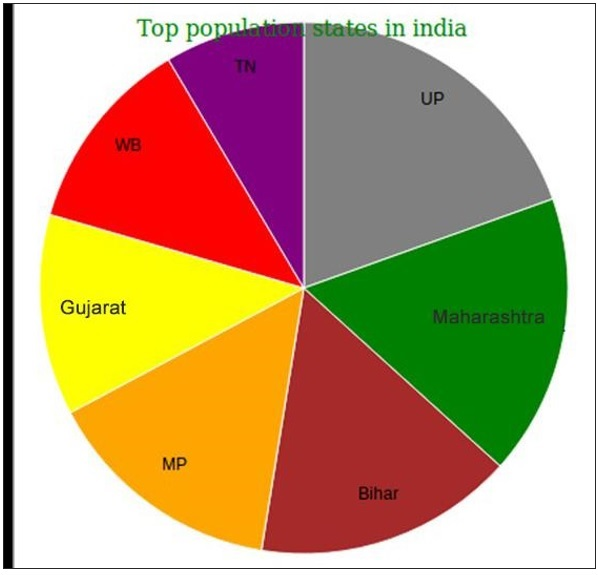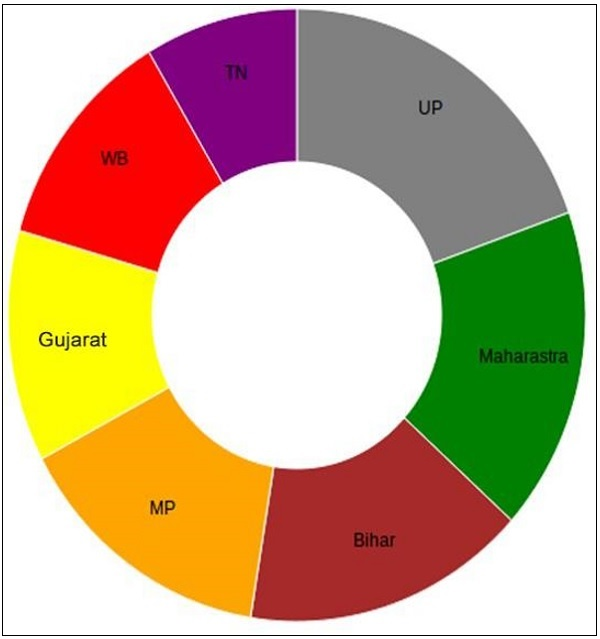# D3.js - 绘制图表

D3.js 用于创建静态 SVG 图表。它有助于绘制以下图表 -

• 条形图
• 圆图
• 饼形图
• 圆环图
• 折线图
• 气泡图等

## 条形图

x 轴（水平轴）表示没有比例的不同类别。y 轴（垂直轴）具有刻度，这表示测量单位。可以垂直或水平绘制条，这取决于类别的数量和类别的长度或复杂性。

### 绘制条形图

svg rect {
fill: gray;
}


svg text {
fill: yellow;
font: 12px sans-serif;
text-anchor: end;
}


<script>
var data = [10, 5, 12, 15];
var width = 300,
scaleFactor = 20,
barHeight = 30;
</script>


• width - SVG 的宽度。

• Scalefactor - 缩放到屏幕上可见的像素值。

• Barheight - 这是水平条的静态高度。

var graph = d3.select("body")
.append("svg")
.attr("width", width)
.attr("height", barHeight * data.length);


var bar = graph.selectAll("g")
.data(data)
.enter()
.append("g")
.attr("transform", function(d, i) {
return "translate(0," + i * barHeight + ")";
});


bar.append("rect")
.attr("width", function(d) {
return d * scaleFactor;
})
.attr("height", barHeight - 1);


bar.append("text")
.attr("x", function(d) { return (d*scaleFactor); })
.attr("y", barHeight / 2)
.attr("dy", ".35em")
.text(function(d) { return d; });


barcharts.html

<html>
<script type = "text/javascript" src = "https://d3js.org/d3.v4.min.js"></script>
<style>
svg rect {
fill: gray;
}

svg text {
fill: yellow;
font: 12px sans-serif;
text-anchor: end;
}
</style>

<body>
<script>
var data = [10, 5, 12, 15];

var width = 300
scaleFactor = 20,
barHeight = 30;

var graph = d3.select("body")
.append("svg")
.attr("width", width)
.attr("height", barHeight * data.length);

var bar = graph.selectAll("g")
.data(data)
.enter()
.append("g")
.attr("transform", function(d, i) {
return "translate(0," + i * barHeight + ")";
});
bar.append("rect").attr("width", function(d) {
return d * scaleFactor;
})

.attr("height", barHeight - 1);

bar.append("text")
.attr("x", function(d) { return (d*scaleFactor); })
.attr("y", barHeight / 2)
.attr("dy", ".35em")
.text(function(d) { return d; });
</script>
</body>
</html>


## 圆图

### 绘制圆图

<script>
var width = 400;
var height = 400;
var data = [10, 20, 30];
var colors = ['green', 'purple', 'yellow'];
</script>


• width - SVG 的宽度。

• height - SVG 的高度。

• 数据 - 数据元素数组。

• 颜色 - 将颜色应用于圆形元素。

var svg = d3.select("body")
.append("svg")
.attr("width", width)
.attr("height", height);


var g = svg.selectAll("g")
.data(data)
.enter()
.append("g")
.attr("transform", function(d, i) {
return "translate(0,0)";
})


var g = svg.selectAll（g - 创建用于保持圆圈的组元素。

.data(data) - 将我们的数据数组绑定到组元素。

.enter() - 为我们的组元素创建占位符。

.append（g - 将组元素添加到我们的页面。

.attr("transform", function(d, i) {
return "translate(0,0)";
})


g.append("circle")


.attr("cx", function(d, i) {
return i*75 + 50;
})


.attr("cy", function(d, i) {
return 75;
})


.attr("r", function(d) {
return d*1.5;
})


.attr("fill", function(d, i){
return colors[i];
})


g.append("text")
.attr("x", function(d, i) {
return i * 75 + 25;
})
.attr("y", 80)
.attr("stroke", "teal")
.attr("font-size", "10px")
.attr("font-family", "sans-serif")
.text(function(d) {
return d;
});


circlecharts.html

<html>
<script type = "text/javascript" src = "https://d3js.org/d3.v4.min.js"></script>

<body>
<script>
var width = 400;

var height = 400;

var data = [10, 20, 30];

var colors = ['green', 'purple', 'yellow'];

var svg = d3
.select("body")
.append("svg")
.attr("width", width)
.attr("height", height);

var g = svg.selectAll("g")
.data(data)
.enter()
.append("g")
.attr("transform", function(d, i) {
return "translate(0,0)";
})

g.append("circle").attr("cx", function(d, i) {
return i*75 + 50;
})

.attr("cy", function(d, i) {
return 75;
})

.attr("r", function(d) {
return d*1.5;
})

.attr("fill", function(d, i){
return colors[i];
})

g.append("text").attr("x", function(d, i) {
return i * 75 + 25;
})

.attr("y", 80)
.attr("stroke", "teal")
.attr("font-size", "10px")
.attr("font-family", "sans-serif").text(function(d) {
return d;
});
</script>
</body>
</html>


## 饼形图

### 画一个饼图

• d3.arc() 方法和
• d3.pie() 方法。

d3.arc() 方法 - d3.arc() 方法生成一个弧。你需要为弧设置内半径和外半径。如果内半径为 0，则结果将为饼图，否则结果将为圆环图，将在下一节中讨论。

d3.pie() 方法 - d3.pie() 方法用于生成饼图。它从数据集中获取数据并计算饼图的每个楔形的起始角度和结束角度。

.arc text {
font: 12px arial;
text-anchor: middle;
}

.arc path {
stroke: #fff;
}

.title {
fill: green;
font-weight: italic;
}


<script>
var svg = d3.select("svg"),
width = svg.attr("width"),
height = svg.attr("height"),
radius = Math.min(width, height) / 2;
</script>


• width - SVG 的宽度。

• height - SVG 的高度。

• radius - 可以使用 Math.min(width，height)/ 2 的函数计算;

var g = svg.append("g")
.attr("transform", "translate(" + width / 2 + "," + height / 2 + ")");


var color = d3.scaleOrdinal(['gray', 'green', 'brown', 'orange']);


var pie = d3.pie()
.value(function(d) { return d.percent; });


var arc = d3.arc()


var label = d3
.arc()


d3.csv("populations.csv", function(error, data) {
if (error) {
throw error;
}
});


var arc = g.selectAll(".arc")
.data(pie(data))
.enter()
.append("g")
.attr("class", "arc");


arcs.append("path")
.attr("d", arc)
.attr("fill", function(d) { return color(d.data.states); });


arc.append("text")
.attr("transform", function(d) {
return "translate(" + label.centroid(d) + ")";
})
.text(function(d) { return d.data.states; });


svg.append("g")
.attr("transform", "translate(" + (width / 2 - 120) + "," + 20 + ")")
.append("text")
.text("Top population states in india")
.attr("class", "title")


population.csv

states,percent
UP,80.00
Maharastra,70.00
Bihar,65.0
MP,60.00
Gujarat,50.0
WB,49.0
TN,35.0


<!DOCTYPE html>
<html>
<style>
.arc text {
font: 12px arial;
text-anchor: middle;
}

.arc path {
stroke: #fff;
}

.title {
fill: green;
font-weight: italic;
}
</style>

<script type = "text/javascript" src = "https://d3js.org/d3.v4.min.js"></script>

<body>
<svg width = "400" height = "400"></svg>
<script>
var svg = d3.select("svg"),
width = svg.attr("width"),
height = svg.attr("height"),
radius = Math.min(width, height) / 2;

var g = svg.append("g")
.attr("transform", "translate(" + width / 2 + "," + height / 2 + ")");

var color = d3.scaleOrdinal([
'gray', 'green', 'brown', 'orange', 'yellow', 'red', 'purple'
]);

var pie = d3.pie().value(function(d) {
return d.percent;
});

var path = d3.arc()

var label = d3.arc()

d3.csv("populations.csv", function(error, data) {
if (error) {
throw error;
}

var arc = g.selectAll(".arc")
.data(pie(data))
.enter()
.append("g")
.attr("class", "arc");

arc.append("path")
.attr("d", path)
.attr("fill", function(d) { return color(d.data.states); });

console.log(arc)

arc.append("text").attr("transform", function(d) {
return "translate(" + label.centroid(d) + ")";
})

.text(function(d) { return d.data.states; });
});

svg.append("g")
.attr("transform", "translate(" + (width / 2 - 120) + "," + 20 + ")")
.append("text").text("Top population states in india")
.attr("class", "title")
</script>
</body>
</html>## 圆环图

var arc = d3.arc()


### Donutchart.html

<!DOCTYPE html>
<html>
<style>
.arc text {
font: 12px arial;
text-anchor: middle;
}

.arc path {
stroke: #fff;
}

.title {
fill: green;
font-weight: italic;
}
</style>
<script type = "text/javascript" src = "https://d3js.org/d3.v4.min.js"></script>

<body>
<svg width = "400" height = "400"></svg>
<script>
var svg = d3.select("svg"),
width = svg.attr("width"),
height = svg.attr("height"),
radius = Math.min(width, height) / 2;

var g = svg.append("g")
.attr("transform", "translate(" + width / 2 + "," + height / 2 + ")");

var color = d3.scaleOrdinal([
'gray', 'green', 'brown', 'orange', 'yellow', 'red', 'purple'
]);

var pie = d3.pie().value(function(d) {
return d.percent;
});

var path = d3.arc()

var label = d3.arc()

d3.csv("populations.csv", function(error, data) {
if (error) {
throw error;
}

var arc = g.selectAll(".arc")
.data(pie(data))
.enter()
.append("g")
.attr("class", "arc");
arc.append("path")
.attr("d", path)
.attr("fill", function(d) { return color(d.data.states); });

console.log(arc)

arc.append("text")
.attr("transform", function(d) {
return "translate(" + label.centroid(d) + ")";
})
.text(function(d) { return d.data.states; });
});

svg.append("g")
.attr("transform", "translate(" + (width / 2 - 120) + "," + 20 + ")")
.append("text")
.attr("class", "title")
</script>
</body>
</html>


var path = d3.arc()